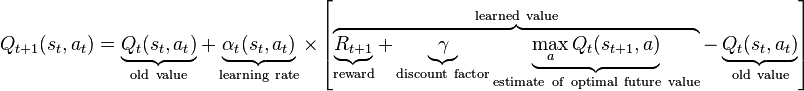2 views

I have implemented Q-Learning as described in,

http://web.cs.swarthmore.edu/~meeden/cs81/s12/papers/MarkStevePaper.pdf

In order to approx. Q(S, A) I use a neural network structure like the following,

• Activation sigmoid

• Inputs, number of inputs + 1 for Action neurons (All Inputs Scaled 0-1)

• Outputs, single output. Q-Value

• N number of M Hidden Layers.

• Exploration method random 0 < rand() < propExplore

At each learning iteration using the following formula,I calculate a Q-Target value then calculate an error using,

error = QTarget - LastQValueReturnedFromNN

and backpropagate the error through the neural network.

Q1, Am I on the right track? I have seen some papers that implement an NN with one output neuron for each action.

Q2, My reward function returns a number between -1 and 1. Is it ok to return a number between -1 and 1 when the activation function is sigmoid (0 1)

Q3, From my understanding of this method given enough training instances it should be quarantined to find an optimal policy wight? When training for XOR sometimes it learns it after 2k iterations sometimes it won't learn even after 40k 50k iterations.

by (108k points)

Answer to your 1st question, the neural network is trained by comparing the output to a target for a given set of inputs and generating an error value. This error is then used to update the connections and/or the weight of those connections in the neural net.

The answer to your second question is that the reward is defined in terms of the task to be achieved. The positive reward is given for successfully achieving the task or for any action that brings the agent closer to solving the task while the negative reward is given for any actions that impede the agent from successfully achieving the task. Sigmoid is typically used for classification.

The answer to your third question is that the results in your referred paper also show that the more complex the environment gets, the better the neural net implementation does over the q-table implementation. We anticipate that the difference between the two approaches in the complex environment might only become apparent after more than 30000 learning iterations.